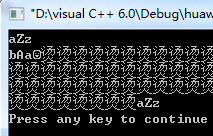//给定一个字符串，把字符串内的字母转换成该字母的下一个字母，a换成b，z换成a，Z换成A，
// 如aBf转换成bCg，字符串内的其他字符不改变，给定函数，
//编写函数 void Stringchang（const char*input,char*output） 其中input是输入字符串，output是输出字符串
#include
#include
void stringchang(const char*input,char*output)
{
for(int i=0;i<255;i++)
{
output[i]=input[i]+1;
switch(input[i])
{
case'z':output[i]='a';break;
case'Z':output[i]='A';break;
}
if(input[i]=='\0')
break;
}

}

void main()
{
char input;
char output;
gets(input);
stringchang(input,output);
puts(output);
}4个回答

#include

void stringchang(const char*input,char*output)
{
for(int i=0;i<255&&input[i]!='\0';i++)
{
output[i]=input[i]+1;
switch(input[i])
{
case'z':output[i]='a';break;
case'Z':output[i]='A';break;
}

}

}
void main()
{
char input={'\0'};
char output={'\0'};
gets(input);
stringchang(input,output);
puts(output);
}